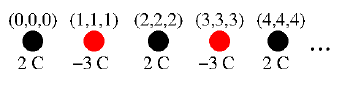# Can you help with this?

조회 수: 5(최근 30일)
Yigit Baran Aydin 9 May 2020
댓글: Rena Berman 14 May 2020There are n point charges in the system and the electrostatic charges of the points are as in the figure. The positions of the points are also shown.
Write a MATLAB script that assigns the loads to the points as shown in the illustration.
Write a MATLAB script that assigns the positions of the points.

#### 댓글 수: 2

Stephen Cobeldick 12 May 2020
Original question from Yigit Baran Aydin, recovered from Google Cache:
Can you help with this?There are n point charges in the system and the electrostatic charges of the points are as in the figure. The positions of the points are also shown.
Write a MATLAB script that assigns the loads to the points as shown in the illustration.
Write a MATLAB script that assigns the positions of the points.
Rena Berman 14 May 2020

### 채택된 답변

Image Analyst 9 May 2020
Try plot() and text(). Here's a start
plot(x, y, 'r.', 'MarkerSize', 50); % or 'k.' for black.
grid on;
caption = sprintf('(%d, %d, %d)', x, x, x);
text(x, y+ 0.2, caption